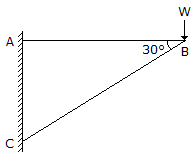Last updated: August 14, 2020

1. The slope on the road surface generally provided on the curves is known as
2. The term 'Centroid' is
3. The acceleration of a body sliding down an inclined surface is
4. Two forces are acting at an angle of 120°. The bigger force is 40N and the resultant is perpendicular to the smaller one. The smaller force is
5. A ladder is resting on a rough ground and leaning against a smooth vertical wall. The force of friction will act
6. Joule is the unit of
7. The negative acceleration is called retardation.
8. A ladder is resting on a smooth ground and leaning against a rough vertical wall. The force of friction will act
9. A force acting in the opposite direction to the motion of the body is called force of friction.
10. When a body of mass 'm' attains a velocity 'v' from rest in time 't', then the kinetic energy of translation is
11. If the efficiency of a lifting machine is kept constant, its velocity ratio is __________ proportional to its mechanical advantage.
12. In a framed structure, as shown in the below figure, the forces in the members AB and BC are respectively13. The length of a second's pendulum is
14. The moment of inertia of a square of side (a) about an axis through its centre of gravity is
15. D' Alembert's principle basically depends upon Newton's second law of motion.
16. Coplaner non-concurrent forces are those forces which __________ at one point, but their lines of action lie on the same plane.
17. For any system of coplaner forces, the condition of equilibrium is that the
18. The moment of inertia of a thin rod of mass 'm' and length 'l', about an axis through its centre of gravity and perpendicular to its length is
19. The velocity ratio of a simple wheel and axle with 'D' and 'd' as the diameters of effort wheel and load axle, is
20. The wheels of a moving car possess
Engineering Mechanics MCQ 13
You got {{userScore}} out of {{maxScore}} correct
{{title}}
{{image}}
{{content}}